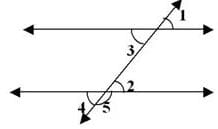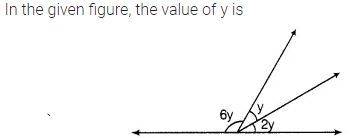# Test: Lines and Angles

## 15 Questions MCQ Test Online MCQ Tests for Class 7 | Test: Lines and Angles

Description
Attempt Test: Lines and Angles | 15 questions in 10 minutes | Mock test for Class 7 preparation | Free important questions MCQ to study Online MCQ Tests for Class 7 for Class 7 Exam | Download free PDF with solutions
QUESTION: 1

### If two lines intersect at a point, then the vertically opposite angles are always ________

Solution:

If two lines intersect at a point, then the vertically opposite angles are always equal

QUESTION: 2

### Two angles forming a linear pair are ________________.

Solution:

Two angles forming a linear pair are supplementary

QUESTION: 3

### A line that intersects two or more lines at distinct points is called

Solution:

A line that intersects two or more lines at distinct points is called transversal

QUESTION: 4

If two adjacent angles are supplementary, then they form _________ .

Solution:

A linear pair is two angles that are adjacent and whose non-common sides form a straight line. If two angles are a linear pair, then they are supplementary.

QUESTION: 5

If two angles are supplementary then the sum of their measures is __________.

Solution:

If two angles are supplementary then the sum of their measures is 180°

QUESTION: 6

If two angles are complementary, then the sum of their measures is __________.

Solution:

If two angles are complementary, then the sum of their measures is 90°.

QUESTION: 7

If ∠S and 100° form a linear pair. What is the measure of ∠S

Solution:

∠S and 100° form a linear pair i.e,

∠S + 100° = 180°

∠S = 180deg - 100°

∠S = 80°

QUESTION: 8

In figure pair of alternate interior angles are :Solution:

Alternate interior angles are formed when a transversal passes through two lines. The angles that are formed on opposite sides of the transversal and inside the two lines are alternate interior angles. The theorem says that when the lines are parallel, that the alternate interior angles are equal.

So in the given figure ∠2, ∠3 are alternate interior angles

QUESTION: 9

Find the measure of an angle, if 7 times its complement is 10 less than 3 times its supplement

Solution:

Let the angle be x
measure of its complement = (90-x)
measure of its supplement= (180-x)
7(90-x) =3(180-x)-10

630 - 7x=540x-3x -10

-7x+3x= 530-630
-4x=-100

x= 100/4
x=25

QUESTION: 10

Find the measure of angle which is 32 less than its supplement

Solution:

Let the supplement angle be x
given:
(x-32) + x = 180
2x = 212
x= 106
The supplement angle is 106

so 32 less than it will be 74

QUESTION: 11

The angle which makes a linear pair with an angle of 61deg is of

Solution:

Linear pair has a sum of 180 degrees
So x+61 =180
x=180 - 61 =119 degrees

QUESTION: 12

The sum of all angles around a point is

Solution:

The sum of all angles around a point is 360deg

QUESTION: 13

If  two lines are intersected by a transversal such that any pair of corresponding angles are equal then the lines are

Solution:

If  two lines are intersected by a transversal such that any pair of corresponding angles are equal then the lines are parallel

QUESTION: 14Solution:

Its a straight angle which is equal to 180 degrees.
So ,6y+y+2y = 180
9y = 180
y= 180/9 = 20 degrees

QUESTION: 15

A and B are vertically opposite angles. If the measure of A is 60o, what is the value of B?

Solution:

When two lines intersect, then vertically opposite angles so formed are equalUse Code STAYHOME200 and get INR 200 additional OFF Use Coupon Code